# Semiconductor Junction Structures

This is a continuation from the previous tutorial - spontaneous emission in semiconductors.

A semiconductor junction device can have either a homostructure or a heterostructure.

A basic homostructure simply consists of a p-n homojunction.

There are a number of different heterostructures, but the two basic concepts are the single heterostructure (SH), which consists of a single heterojunction, and the double heterostructure (DH), which consists of two heterojunctions.

When the layer between the junctions of a DH is thin enough, the structure becomes a quantum well (QW) because of the quantum size effect in the thin layer.

An electrically pumped LED or semiconductor laser has the basic structure of a semiconductor diode. In normal operation, an LED or semiconductor laser is pumped under forward bias by electric current injection to create an excess concentration of electron-hole pairs in an active layer, or an active region, where radiative recombination takes place. For this reason, electrically pumped semiconductor lasers are also called laser diodes, diode lasers, or injection lasers.

A practical LED can have either a homostructure or a heterostructure, but all practical semiconductor lasers are made of heterostructures for the significant advantages of semiconductor heterostructures over homostructures in terms of carrier confinement and optical waveguiding.

Tight carrier confinement creates a high carrier concentration for a given injection current; tight optical confinement makes stimulated emission efficient. There are two critical issues in lowering the threshold and in improving the efficiency of a semiconductor laser.

Because of the many advantages of quantum wells, many semiconductor lasers are quantum-well lasers consisting of a single quantum well (SQW) or multiple quantum wells (MQW).

The optical field in an LED or a semiconductor laser can be either horizontally propagating, in a direction parallel to the junction plane, or vertically propagating, in a direction perpendicular to the junction plane.

An LED or semiconductor can be either edge emitting or surface emitting.

The optical wave in an edge-emitting device propagates horizontally and is emitted from one or two side surfaces of the structure that are perpendicular to the junction plane.

The optical wave in a surface-emitting device can propagate either vertically or horizontally in the structure, but it is emitted from a surface that is parallel to the junction interface.

Various structures for edge-emitting and surface-emitting devices are discussed in later tutorials.

## Homostructures

The characteristics of a homostructure device under forward bias are shown in Figure 13-10.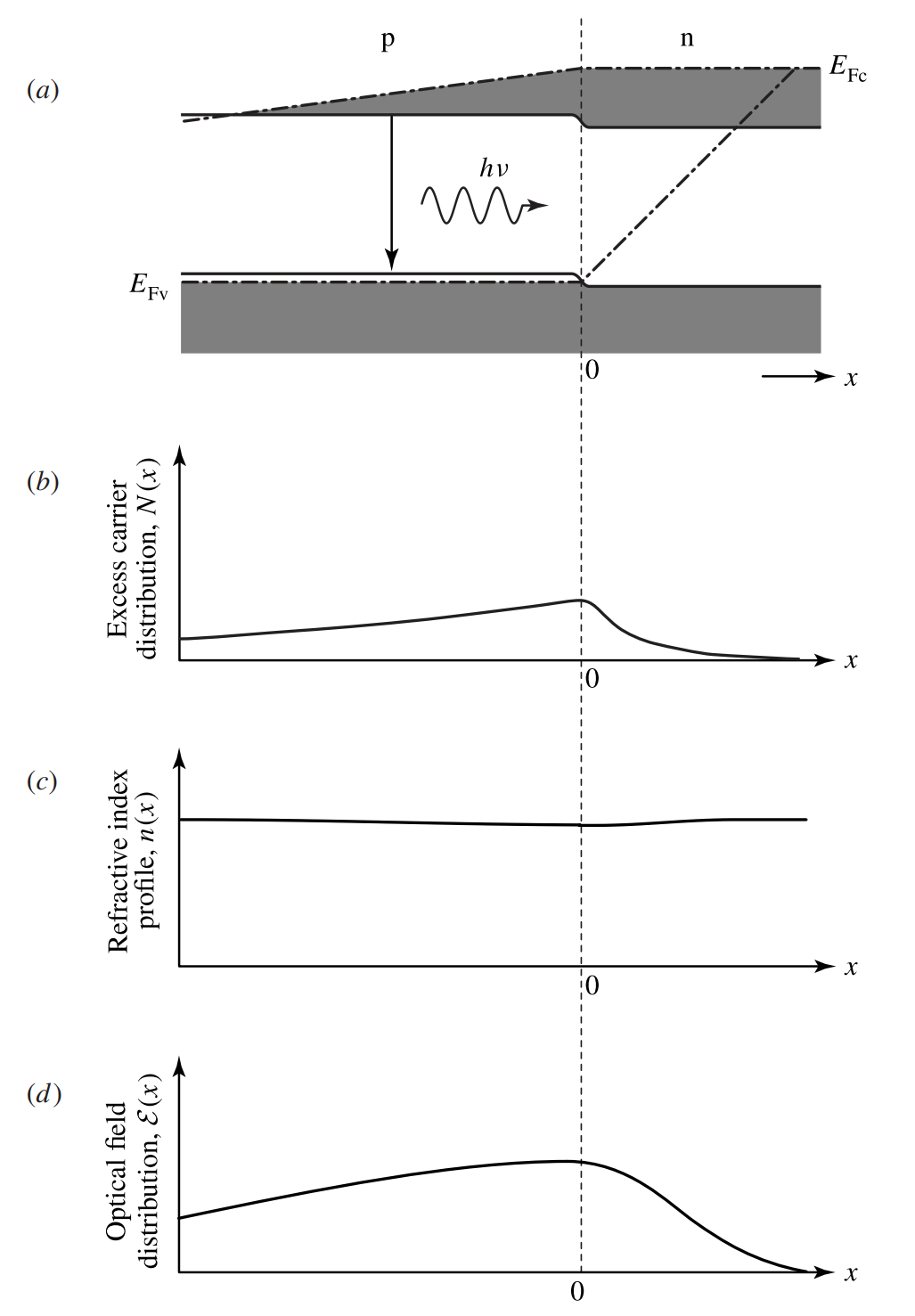Figure 13-10.  (a) Energy bands, (b) excess carrier distribution, (c) refractive index profile, and (d) distribution of a horizontally propagating optical field of a p-n homostructure device under forward bias.

Usually both p and n regions of a homostructure device are heavily doped for good conductivity throughout the device.

The excess minority electrons on the p side due to electron injection from the n side are distributed over an electron diffusion length of $$L_\text{e}$$, and the excess minority holes on the n side due to hole injection from the p side are distributed over a hole diffusion length of $$L_\text{h}$$.

In a given semiconductor, $$D_\text{e}\gg{D}_\text{h}$$ because the electron mobility $$\mu_\text{e}$$ is generally higher than the hole mobility $$\mu_\text{h}$$.

Then, according to (12-114) [refer to the semiconductor junctions tutorial], if the p side is not much more heavily doped than the n side, the injection current is predominantly carried by the electrons injected from the n side to the p side.

If the injected minority electrons in the p region and the injected minority holes in the n region have comparable lifetimes, as is normally the case in the operating conditions of LEDs and lasers, that fact that $$D_\text{e}\gg{D}_\text{h}$$ also implies that $$L_\text{e}\gg{L}_\text{h}$$.

Therefore, the total number of excess minority electrons on the p side is much larger than that of excess minority holes on the n side.

As expressed in (12-55) [refer to the carrier recombination in semiconductors tutorial], (12-107) and (12-108) [refer to the semiconductor junctions tutorial], in normal operating conditions of LEDs and semiconductor lasers, excess electrons and holes appear as excess electron-hole pairs of equal concentration $$N(x)$$ at any given location $$x$$.

From the above discussions, we find that the excess carriers in a homostructure LED or semiconductor laser are distributed mainly in the p region of the device over an electron diffusion length $$L_\text{e}$$ from the junction, as illustrated in Figure 13-10(b).

For an LED, this region is the active layer where radiative carrier recombination for light emission takes place. For a semiconductor laser, an optical gain exists at the locations where $$N(x)\gt{N}_\text{tr}$$ within this active layer.

In a homostructure device, the transverse distribution of a horizontally propagating optical field and the distribution of the excess carriers overlap.

There is slight variation of the refractive index in the vicinity of the junction due to spatial variation of the carrier density, but it has little waveguiding effect on the optical field.

Therefore, the optical field in a homostructure device is not well confined in the vertical direction but spreads over a distance that can be as large as a few times $$L_\text{e}$$.

These characteristics are illustrated in Figure 13-10(c) and (d).

Clearly, a homostructure has two major deficiencies:

1. The excess carriers are neither confined nor concentrated but are spread by diffusion. For this reason, the thickness of the active layer in a homostructure is normally on the order of one to a few micrometers, depending on the diffusion length of the electrons.
2. There is no waveguiding mechanism in the structure for optical confinement. It is therefore difficult to control the spatial mode characteristics of a homostructure laser diode.

Both problems can be solved with properly designed heterostructures.

## Single Heterostructures

Because the distribution of the excess carriers in a homostructure is largely determined by the diffusion of the minority electrons in the p region, it is possible to place a P-p heterojunction in the p region to restrict the diffusion of excess electrons that are injected into the p region across the p-n junction. This additional P-p heterojunction results in a P-p-n single heterostructure (SH) diode, the characteristics of which under forward bias are illustrated in Figure 13-11.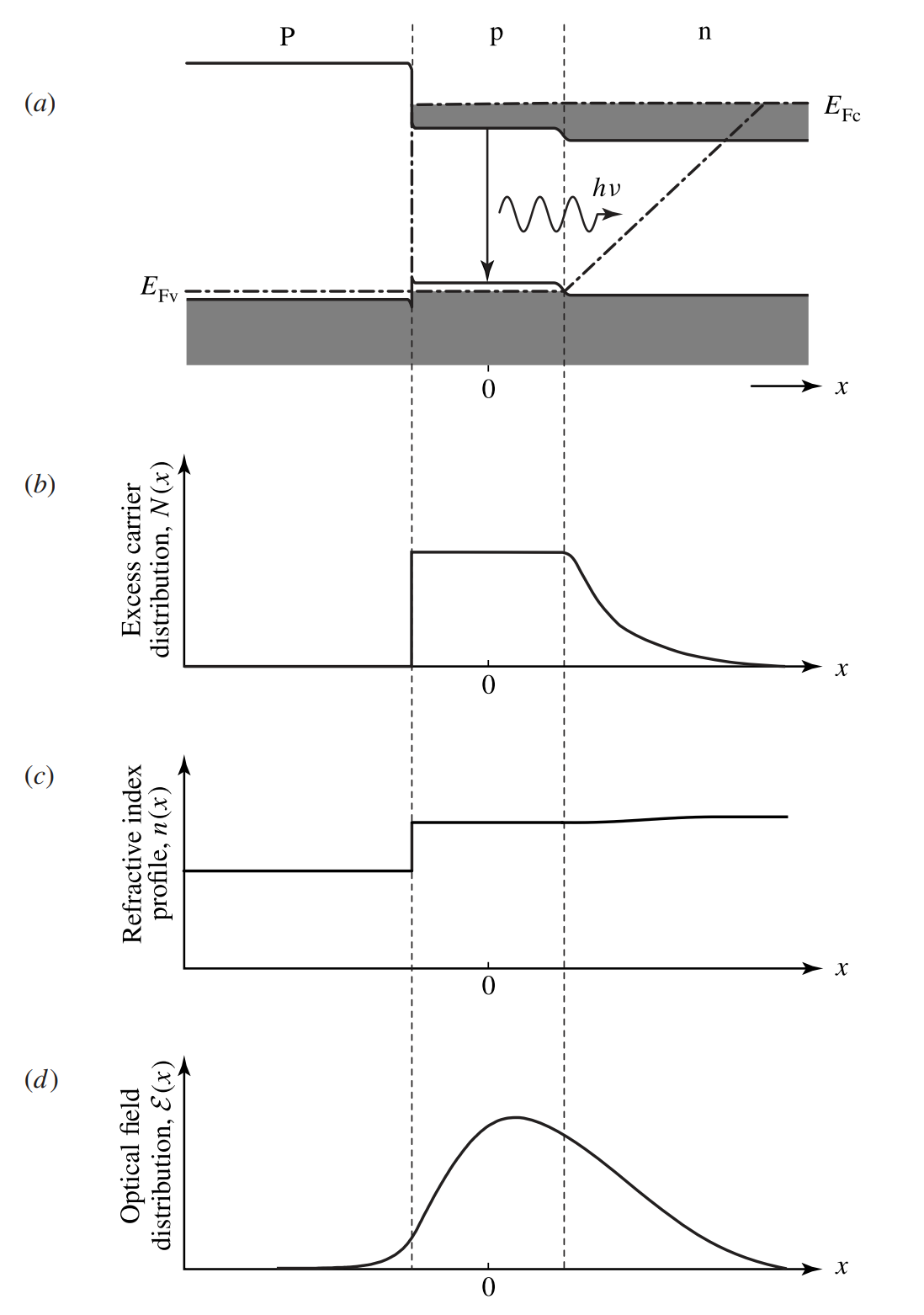Figure 13-11.  (a) Energy bands, (b) excess carrier distribution, (c) refractive index profile, and (d) distribution of a horizontally propagating optical field of a P-p-n single heterostructure device under forward bias.

The n region of a P-p-n SH is heavily doped so that electron injection into the p region completely dominates hole injection into the n region.

As illustrated in Figure 13-11(b), the energy barrier at the P-p heterojunction blocks the diffusion of injected electrons so that they are confined within the narrow-gap p region, which thus defines an active layer populated with excess carriers.

The thickness of this active layer can be controlled by the location of the P-p heterojunction with respect to that of the p-n junction.

A heterostructure is fabricated with lattice-matched layers of compound semiconductors that have different compositions.

One important feature of such materials is that at a given optical wavelength, the refractive index of a narrow-gap composition is higher than that of a wide-gap composition.

Therefore, in addition to carrier confinement, the heterojunction also provides the needed index step, shown in Figure 13-11(c), for optical confinement of a horizontally propagating optical field.

However, because no significant index step appears at the p-n junction, optical confinement in the SH geometry is one-sided and is not completely effective, as illustrated in Figure 13-11(d).

## Double Heterostructures

Very effective carrier and optical confinement can be simultaneously accomplished with DH geometry. A basic DH can be either P-p-N or P-n-N. Figure 13-12 shows the basic characteristics of a P-p-N DH under forward bias.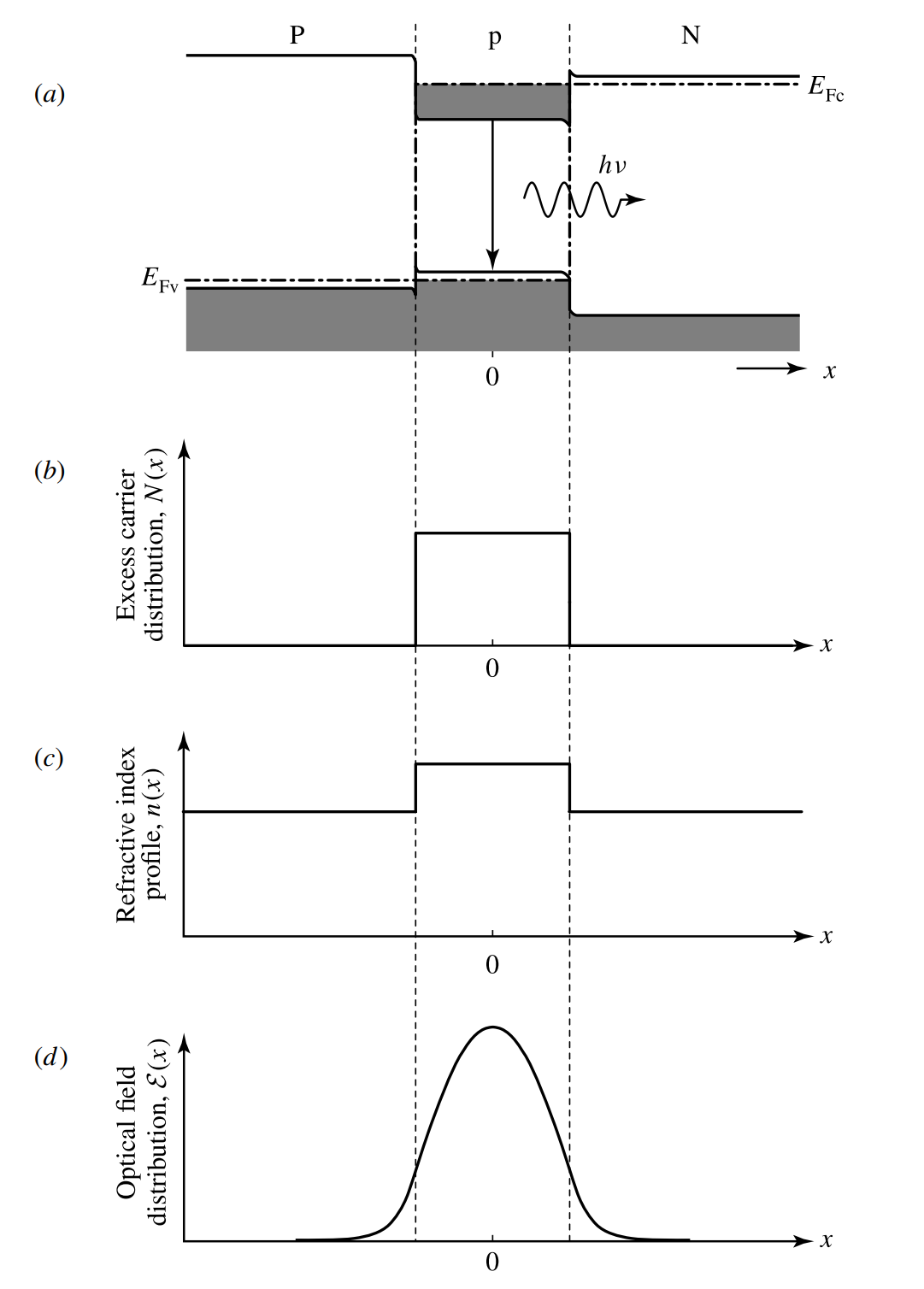Figure 13-12.  (a) Energy bands, (b) excess carrier distribution, (c) refractive index profile, and (d) distribution of a horizontally propagating optical field of a P-p-N double heterostructure under forward bias.

From the discussions in the semiconductor junctions tutorial regarding carrier injection across a heterojunction, we know that the injection current in a P-p-N structure is primarily carried by the electrons injected from the wide-gap N region to the narrow-gap p region, whereas the injection of holes from the P region to the n region dominates in a P-n-N structure.

In either structure, almost all of the excess carriers created by current injection are injected into the narrow-gap active layer and are confined within this layer by the energy barriers of the heterojunctions on both sides of the active layer, as illustrated in Figure 13-12(b) for a P-p-N structure.

Because the narrow-gap active layer has a higher refractive index than the wide-gap outer layers on both sides, an optical waveguide with the active layer being the waveguide core is built into the DH geometry for vertical confinement of a horizontally propagating optical field, as shown in Figures 13-12(c) and (d).

Because of the index steps on both sides of the active layer, the waveguide in the DH geometry is much more effective than that in the SH geometry. The active layer in a DH device is typically in the range of 100-300 nm. By properly designing the structure with sufficient index steps, the waveguiding active layer in a DH can be made as thin as 100 nm or less while maintaining effective confinement of the optical wave.

Compared to the active layer of a homostructure that has a thickness of the order of 1 μm or larger, which is determined by electron diffusion, the confinement of carriers in a thin DH active layer greatly increases the concentration of excess carriers at a given injection level, resulting in a high radiative efficiency for a DH LED and a large optical gain for a DH semiconductor laser.

One additional advantage of a DH device is that because the optical radiation in the device has a photon energy near the narrow bandgap of the active layer, the wide-gap outer layers are transparent to the optical wave in the device, thus reducing the absorption loss of the device in comparison to that of a homostructure or SH device.

Except for some specially designed devices in which intentional strain caused by lattice mismatch is desired, common DH devices are fabricated with lattice-matched layers to reduce the defects and the recombination centers at the heterojunction interfaces so that good current injection efficiency and high radiative efficiency can both be obtained.

## Quantum-Well Structures

In terms of structure, a quantum well is just a very thin DH.

As the active layer of a semiconductor DH gets thinner than about 50 nm, the effect of quantum confinement for the electrons and holes in the thin active layer starts to appear in the direction perpendicular to the junction plane.

This effect leads to quantization of momentum in the perpendicular direction, resulting in discrete energy levels associated with the motion of electrons and holes in this direction, as shown in Figure 13-13.

In the horizontal dimensions, electrons and holes remain free and form energy bands. As a result, both conduction and valence bands are split into a number of subbands corresponding to the quantized levels, as shown in Figure 13-13.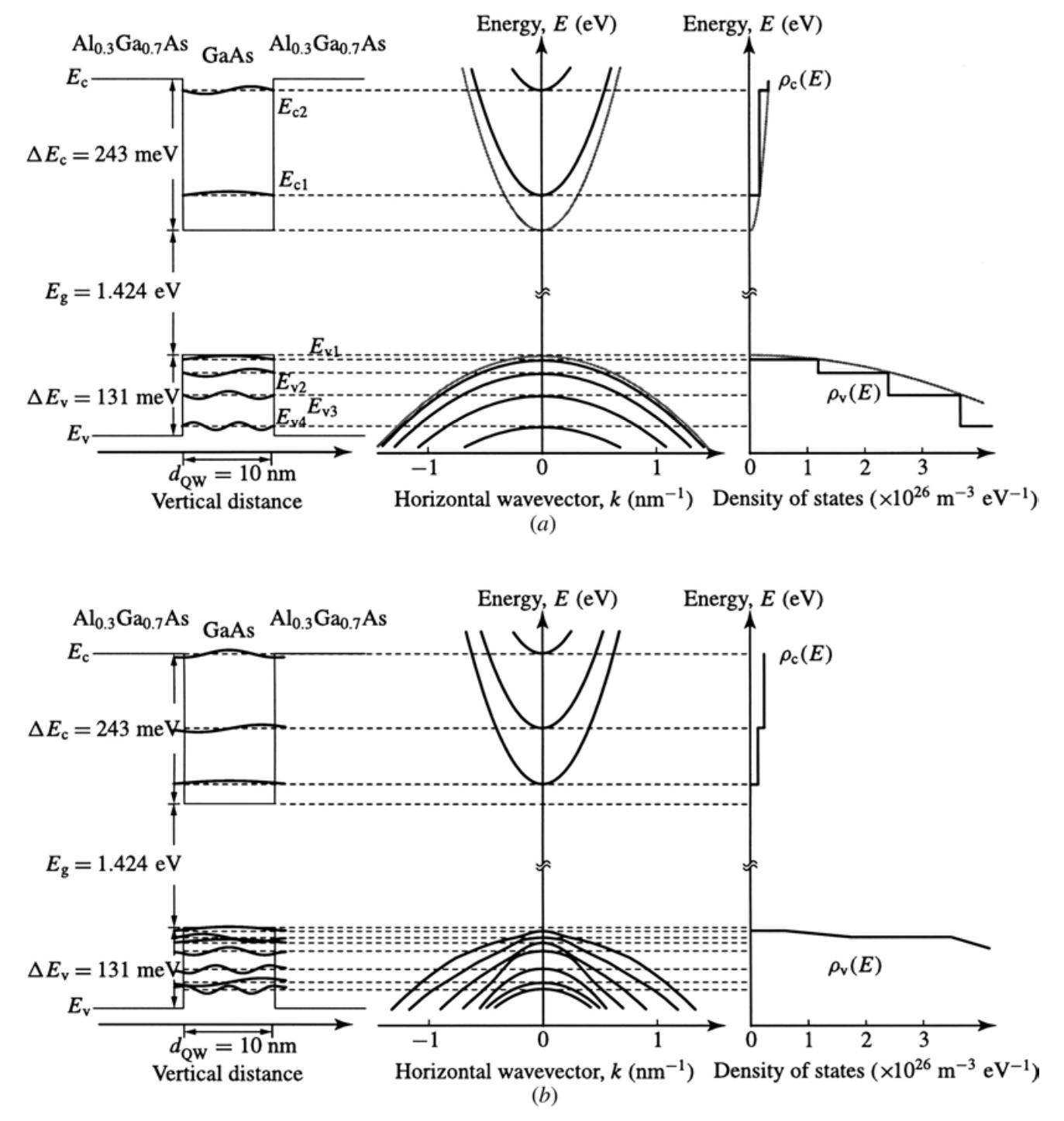Figure 13-13.  Quantized energy levels and corresponding subbands of a semiconductor quantum well. Shown as a quantitative example here is a square Al0.3Ga0.7As/GaAs/Al0.3Ga0.7As quantum well at $$300\text{ K}$$ that has a thickness of $$d_\text{QW}=10\text{ nm}$$. Shown in (a) are simplified, but not completely realistic, characteristics calculated using the quantized states of infinite wells as approximation. The conduction band is plotted for $$m_\text{e}^*=0.067m_0$$, and the valence band is plotted for $$m_\text{h}^*=0.52m_0$$ by combining the heavy- and light-hole bands into one band. Thus, separate quantized levels of heavy and light holes are not shown. The gray curves represent bulk properties of GaAs. Shown in (b) are characteristics obtained by considering finite well heights, separate heavy- and light-hole bands, and interaction among the quantized hole subbands.

For a particle of an effective mass $$m^*$$ in an infinite square quantum well of a width $$d_\text{QW}$$, the energies of the quantized levels are inversely proportional to $$m^*d_\text{QW}^2$$ but increase quadratically with an integral quantum number $$q$$, which starts with $$q=1$$ for the lowest quantized level.

A semiconductor quantum well is not an infinite potential well because the heights of the energy steps at the DH junctions are finite. However, taking the energy quantization of an infinite potential well as an approximation, we can express the band edges of the quantized conduction and valence subbands, respectively, as

$\tag{13-49}E_\text{c,q}^\text{QW}=E_\text{c}+\frac{q^2h^2}{8m_\text{e}^*d_\text{QW}^2}$

and

$\tag{13-50}E_\text{v,q}^\text{QW}=E_\text{v}-\frac{q^2h^2}{8m_\text{h}^*d_\text{QW}^2}$

where $$q=1,2,3\ldots$$.

The number of quantized subbands for electrons and that for holes depend on the heights of the potential barriers, $$\Delta{E}_\text{c}$$ in the conduction band and $$\Delta{E}_\text{v}$$ in the valence band, as well as on the well width $$d_\text{QW}$$.

The effective bandgap for a quantum well is no longer $$E_\text{g}$$ of the semiconductor material in the active layer but is the separation between the lowest subband of the conduction band and the highest subband of the valence band, both associated with the $$q=1$$ quantized levels.

The selection rules for optical transitions of electrons between quantized conduction subbands and quantized valence subbands require that only transitions between a conduction subband and a valence subband of the same quantum number $$q$$ are allowed.

Thus, optical transitions take place only between the $$q=1$$ conduction subband and the $$q=1$$ valence subband or between the $$q=2$$ conduction subband and the $$q=2$$ valence subband, and so on, but not between the $$q=1$$ conduction subband and the $$q=2$$ valence subband or between the $$q=2$$ conduction subband and the $$q=1$$ valence subband, and so forth.

The photon energy required for transition between the conduction subband of quantum number $$q$$ and the valence subband of quantum number $$q$$ is

$\tag{13-51}h\nu\gt{E}_\text{g}+\frac{q^2h^2}{8m_\text{e}^*d_\text{QW}^2}+\frac{q^2h^2}{8m_\text{h}^*d_\text{QW}^2}=E_\text{g}+\frac{q^2h^2}{8m_\text{r}^*d_\text{QW}^2}$

where $$m_\text{r}^*$$ is the reduced effective mass defined in (13-15) [refer to the band-to-band optical transitions in semiconductors tutorial].

Clearly, the lowest photon energy required for a transition between the conduction band and the valence band of a quantum well is that with $$q=1$$ in (13-51). Therefore, a quantum well has an effective bandgap given by

$\tag{13-52}E_\text{g}^{QW}=E_\text{g}+\frac{h^2}{8m_\text{e}^*d_\text{QW}^2}+\frac{h^2}{8m_\text{h}^*d_\text{QW}^2}=E_\text{g}+\frac{h^2}{8m_\text{r}^*d_\text{QW}^2}$

which is larger than that of the bulk semiconductor by an amount that is inversely proportional to the square of the well width.

Example 13-6

A square Al0.3Ga0.7As/GaAs/Al0.3Ga0.7As quantum well has a thickness of $$d_\text{QW}=10\text{ nm}$$, as shown in Figure 13-13.

(a) By approximating the quantization of this quantum well with that of an infinite potential well, find the quantized electron and hole energy levels that define the edges of the conduction and valence subbands, respectively.

(b) By taking band offsets to be $$\Delta{E}_\text{c}\approx65\%\Delta{E}_\text{g}$$ and $$\Delta{E}_\text{v}\approx35\%\Delta{E}_\text{g}$$, find the number of conduction subbands and valence subbands for this finite quantum well.

(c) What are the photon energies and the corresponding optical wavelengths for optical transitions between the conduction and valence subbands?

(d) What is the effective bandgap of this quantum well?

(e) What is the effect of the finite energy height of the quantum well in reality?

(a)

The well region consists of GaAs, which has $$m_\text{e}^*=0.067m_0$$ and $$m_\text{h}^*=0.52m_0$$ from Table 12-2 [refer to the current density in semiconductors tutorial]. The subband edges are defined by the quantized energy levels of the quantum well. Thus, according to (13-49), the conduction subband edges are located at

\begin{align}E_{\text{c}, q}^{QW}-E_\text{c}&=\frac{q^2h^2}{8m_\text{e}^*d_\text{QW}^2}\\&=q^2\times\frac{(6.626\times10^{-34})^2}{8\times0.067\times9.11\times10^{-31}\times(10\times10^{-9})^2}\times\frac{1}{1.6\times10^{-19}}\text{ eV}\\&=56.2q^2\text{ meV},\text{ for }q=1,2,\ldots,\end{align}

and, according to (13-50), the valence subband edges are located at

\begin{align}E_{\text{v}, q}^{QW}-E_\text{v}&=-\frac{q^2h^2}{8m_\text{h}^*d_\text{QW}^2}\\&=-q^2\times\frac{(6.626\times10^{-34})^2}{8\times0.52\times9.11\times10^{-31}\times(10\times10^{-9})^2}\times\frac{1}{1.6\times10^{-19}}\text{ eV}\\&=-7.2q^2\text{ meV},\text{ for }q=1,2,\ldots,\end{align}

(b)

At $$300\text{ K}$$, $$\Delta{E}_\text{g}=374\text{ meV}$$ because $$E_\text{g}=1.424\text{ eV}$$ for GaAs and $$E_\text{g}=1.798\text{ eV}$$ for Al0.3Ga0.7As, according to (12-3) [refer to the introduction to semiconductors tutorial].

This quantum well has an energy height of $$\Delta{E}_\text{c}\approx65\%\Delta{E}_\text{g}=243\text{ meV}$$ in the conduction band and an energy height of $$\Delta{E}_\text{v}\approx35\%\Delta{E}_\text{g}=131\text{ meV}$$ in the valence band.

We then find that the quantum well can only accommodate two conduction subbands because $$3^2\times56.2\text{ meV}=505.8\text{ meV}\gt\Delta{E}_\text{c}=243\text{ meV}\gt2^2\times56.2\text{ meV}=224.8\text{ meV}$$.

We also find that it can accommodate four valence subbands bedcause $$5^2\times7.2\text{ meV}=180\text{ meV}\gt\Delta{E}_\text{v}=131\text{ meV}\gt4^2\times7.2\text{ meV}=115.2\text{ meV}$$.

Thus, there are two conduction subbands with band edges located at 56.2 meV ($$q=1$$) and 224.8 meV ($$q=2$$) from $$E_\text{c}$$ of GaAs, and four valence subbands with band edges located at -7.2 meV ($$q=1$$), -28.8 meV ($$q=2$$), -64.8 meV ($$q=3$$), and -115.2 meV ($$q=4$$) from $$E_\text{v}$$ of GaAs.

These characteristics are shown in Figure 13-13(a).

(c)

The selection rules require that optical transitons occur only between conduction and valence subbands of the same quantum number $$q$$. Though there are four valence subbands, no corresponding conduction subbands are found for $$q=3$$ and $$q=4$$ becausae there are only two conduction subbands.

Therefore, there are only two possible transitions for $$q=1$$ and $$q=2$$, respectively. The photon energy required for the transition between the $$q=1$$ subbands is

$h\nu\gt1.424\text{ eV}+0.0562\text{ eV}+0.0072\text{ eV}=1.4874\text{ eV}$

which corresponds to an optical wavelength of $$\lambda\lt834\text{ nm}$$.

The photon energy required for the transition between the $$q=2$$ subbands is

$h\nu\gt1.424\text{ eV}+0.2248\text{ eV}+0.0288\text{ eV}=1.6776\text{ eV}$

which corresponds to an optical wavelength of $$\lambda\lt739\text{ nm}$$.

(d)

The effective bandgap of the quantum well is determined by the $$q=1$$ conduction- and valence-subband edges. Thus, according to the result found in (c) for the $$q=1$$ transition, the effective bandgap $$E_\text{g}^\text{QW}=1.4874\text{ eV}$$, corresponding to an optical wavelength of $$\lambda_\text{g}^\text{QW}=834\text{ nm}$$.

Compared to the bandgap of $$E_\text{g}=1.424\text{ eV}$$ at $$\lambda_\text{g}=871\text{ nm}$$ for GaAs, the effective bandgap is increased by 63.4 meV with a corresponding blue-shift of the bandgap optical wavelength by 37 nm due to the quantum confinement effect.

(e)

In the above, we have used the formula for the energy levels of an infinite square well to calculate the energies of the subband edges of the quantum well.

In reality, the barrier heights of the quantum well for both conduction and valence bands are finite, being $$\Delta{E}_\text{c}=243\text{ meV}$$ for the conduction band and $$\Delta{E}_\text{v}=131\text{ meV}$$ for the valence band in teh case considered here.

There are two major effects of these finite energy heights.

First, each quantized energy level that defines a subband edge is actually somewhat smaller in its absolute magnitude than what is found above using the formula for an infinite potential well.

Second, because of the first effect, there can be more quantized levels than those obtained above.

A further complication in reality is that fact that there are heavy-  and light-hole bands in the valence band.

For this quantum well, it is found that there are actually three electron subbands, with band edges at 30.1, 117, and 232 meV from $$E_\text{c}$$, in the conduction band, and five heavy-hole subbands, at -4, -14.4, -35.9, -63.6, and -97.5 meV from $$E_\text{v}$$, and two light-hole subbands, at -22.6 and -87.1 meV from $$E_\text{v}$$, in the valence band.

Therefore, the actual bandgap is $$E_\text{g}^\text{QW}=1.4581\text{ eV}$$, which is smaller than $$1.4874\text{ eV}$$ found above, and the bandgap wavelength is $$\lambda_\text{g}^\text{QW}=850\text{ nm}$$, which is longer than 834 nm found above.

The quantized heavy- and light-hole subbands can interact with one another, resulting in mixed subbands due to band crossing.

These characteristics are shown in Figure 13-13(b).

For a fixed well width of $$10\text{ nm}$$, $$E_\text{g}^\text{QW}$$ decreases while $$\lambda_\text{d}^\text{QW}$$ increases correspondingly as the barrier height decreases. This can be accomplished by reding the Al content of the AlGaAs barrier layer to reduce the energy barrier height.

It is a fundamental fact of quantum-mechanical quantization, however, that there is always at least one quantized energy level in a symmetric potential well such as a square well no matter how shallow the well is

Therefore, there are always at least one quantized conduction subband and one quantized valence subband no matter how low the well energy heights in the conduction and valence bands are reduced before they completely vanish.

A very important property of the quantum well is that its density of states is different from that of the bulk semiconductor.

Because of quantization in the perpendicular direction, the density of states for each subband is that of a two-dimensional system divided by the thickness of the well.

Every subband in the conduction band has the same density of states, and every subband in the valence band also has the same density of states:

$\tag{13-53}\rho_\text{c}(E)\text{d}E=\frac{4\pi{m}_\text{e}^*}{h^2d_\text{QW}}\text{d}E,\qquad\rho_\text{v}(E)\text{d}E=\frac{4\pi{m}_\text{h}^*}{h^2d_\text{QW}}\text{d}E$

for each conduction subband and each valence subband, respectively.

For band-to-band optical transitions in a quantum well, (13-13) [refer to the band-to-band optical transitions in semiconductors tutorial] for $$E_2$$ and (13-14) for $$E_1$$ are still valid except that $$E_2-E_1=h\nu$$ has to satisfy the condition in (13-51).

The density of states $$\rho(\nu)$$, defined in (13-16) [refer to the band-to-band optical transitions in semiconductors tutorial], for band-to-band optical transitions in a quantum well is then given by

$\tag{13-54}\rho(\nu)\text{d}\nu=\frac{4\pi{m}_\text{r}^*}{hd_\text{QW}}\sum_qH\left(h\nu-E_\text{g}-\frac{q^2h^2}{8m_\text{r}^*d_\text{QW}^2}\right)\text{d}\nu$

where $$H(x)$$ is the Heaviside function, which has a value of $$H(x)=0$$ for $$x\lt0$$ and a value of $$H(x)=1$$ for $$x\gt0$$.

Clearly, this density of states for optical transitions in a quantum well is different from that given in (13-17) [refer to the band-to-band optical transitions in semiconductors tutorial] for optical transitions in a bulk semiconductor.

In a quantum well, dominant optical transitions occur between the first conduction subband and the first valence subband because those subbands are most populated by electrons and holes, respectively.

The density of states for optical transitions, $$\rho(\nu)$$, obtained in (13-54) is the most significant basic property of a quantum well that sets it apart from bulk semiconductors.

Irrespective of this difference, most of the concepts discussed in the previous four tutorials are valid for quantum wells too.

The relations obtained in those four previous tutorials can also be applied to a quantum well with the modification that $$\rho(\nu)$$ given in (13-54), instead of that in (13-17) [refer to the band-to-band optical transitions in semiconductors tutorial], is used, along with any other modifications that are needed to take account of the relations in (13-51) and (13-53).

For example, the optical gain coefficient $$g(\nu)$$ expressed in (13-31) [refer to the optical gain in semiconductors tutorial] now takes the form:

\tag{13-55}\begin{align}g(\nu)&=\frac{c^2}{8\pi{n}^2\nu^2\tau_\text{sp}}[f_\text{c}(E_2)-f_\text{v}(E_1)]\rho(\nu)\\&=\frac{c^2m_\text{r}^*}{2n^2\nu^2\tau_\text{sp}hd_\text{QW}}[f_\text{c}(E_2)-f_\text{v}(E_1)]\sum_qH\left(h\nu-E_\text{g}-\frac{q^2h^2}{8m_\text{r}^*d_\text{QW}^2}\right)\end{align}

for band-to-band optical transitions in a quantum well.

As another example, the condition for population inversion given in (13-35) [refer to the optical gain in semiconductors tutorial] is now modified to

$\tag{13-56}\Delta{E}_\text{F}=E_\text{Fc}-E_\text{Fv}\gt{h}\nu\gt{E}_\text{g}^\text{QW}=E_\text{g}+\frac{h^2}{8m_\text{r}^*d_\text{QW}^2}$

for a quantum well of a thickness $$d_\text{QW}$$, according to (13-51).

When the condition in (13-56) is first reached, the optical gain coefficient of a quantum well is simply that given in (13-55) for $$q=1$$ because optical transitions only take place between the first conduction subband and the first valence subband.

As the injected carrier density increases to a level at which the population inversion reaches the second conduction and valence subbands, additional gain contributed by transitions between the second subbands adds to the gain that originally exists from the transitions between the first subbands.

If higher subbands exist, further contribution to the gain can come from transitions between higher subbands at higher injection levels.

In contrast, the gain coefficient of a bulk semiconductor increases smoothly with carrier density.

Because a quantum well has a constant two-dimensional density of states, the transparency and gain characteristics of a quantum well are dependent on the two-dimensional carrier density, $$N_\text{2D}=Nd_\text{QW}$$, which is measured per square meter, of the quantum well.

For a given material, the two-dimensional transparency carrier density, $$N_\text{tr}^\text{2D}$$, is a constant that is independent of well width.

Thus, the three-dimensional transparency carrier density is a function of well width:

$\tag{13-57}N_\text{tr}=\frac{N_\text{tr}^\text{2D}}{d_\text{QW}}$

The carrier dependence of the peak gain coefficient of a quantum well is not as linear as that of a bulk semiconductor; it can be approximated as

$\tag{13-58}g_\text{max}(N)=\sigma{N}_\text{tr}\ln\frac{N}{N_\text{tr}}$

where the gain cross section $$\sigma$$ is the differential gain at the transparency carrier density defined as

$\tag{13-59}\sigma=\left.\frac{\text{d}g}{\text{d}N}\right|_{N_\text{tr}}$

The value of $$\sigma$$ is relatively independent of well width. Therefore, the value of $$\sigma{N}_\text{tr}$$ is inversely proportional to well width. For $$N-N_\text{tr}\ll{N}_\text{tr}$$, this relation can be approximated as a linear relation in the form of (13-40) [refer to the optical gain in semiconductors tutorial]:

$\tag{13-60}g_\text{max}(N)=\sigma(N-N_\text{tr})$

Therefore $$\sigma$$ has the meaning of the gain cross section for carrier densities close to the transparency carrier denstiy.

Example 13-7

For GaAs quantum wells, $$N_\text{tr}^\text{2D}=1.16\times10^{16}\text{ m}^{-2}$$ and $$\sigma=2.2\times10^{-19}\text{ m}^2$$. Find the values of $$N_\text{tr}$$ and $$\sigma{N}_\text{tr}$$ for (a) a GaAs quantum well of 10 nm thickness and (b) a GaAs quantum well of 8 nm thickness.

(a)

For $$d_\text{QW}=10\text{ nm}$$, we have

$N_\text{tr}=\frac{N_\text{tr}^\text{2D}}{d_\text{QW}}=\frac{1.16\times10^{16}}{10\times10^{-9}}\text{ m}^{-3}=1.16\times10^{24}\text{ m}^{-3}$

and

$\sigma{N}_\text{tr}=2.2\times10^{-19}\times1.16\times10^{24}\text{ m}^{-1}=2.55\times10^{5}\text{ m}^{-1}$

(b)

For $$d_\text{QW}=8\text{ nm}$$, we have

$N_\text{tr}=\frac{N_\text{tr}^\text{2D}}{d_\text{QW}}=\frac{1.16\times10^{16}}{8\times10^{-9}}\text{ m}^{-3}=1.45\times10^{24}\text{ m}^{-3}$

and

$\sigma{N_\text{tr}}=2.2\times10^{-19}\times1.45\times10^{24}\text{ m}^{-1}=3.19\times10^{5}\text{ m}^{-1}$

We find that the values of $$N_\text{tr}$$ for GaAs quantum wells are not much different from $$N_\text{tr}=1.22\times10^{24}\text{ m}^{-3}$$ found in Example 13-3 [refer to the optical gain in semiconductors tutorial] for bulk GaAs.

In contrast, the value of $$\sigma$$ for GaAs quantum wells is much larger than $$\sigma=3.37\times10^{-20}\text{ m}^{2}$$ found in Example 13-4 [refer to the optical gain in semiconductors tutorial] for bulk GaAs. However, this value of $$\sigma$$ for the quantum wells is evaluated at the transparency carrier density, and it decreases as the carrier density increases high above the transparency density.

Quantum wells have several advantages over bulk semiconductor media. The injected carriers are most concentrated in a quantized subband of a quantum well than in the entire band of a bulk semiconductor.

Because the density of states for each subband of a quantum well is a constant that does not vary with energy, there are already a large number of electrons of the same energy near the edge of a conduction subband and a large number of holes of the same energy near the edge of a valence subband.

In comparison, near the edges of the conduction and valence bands of a bulk semiconductor, there are very few electrons and holes because the density of states in a bulk medium varies with energy and starts at zero from the band edges.

Therefore, a quantum well has a much larger gain cross section $$\sigma$$ than a bulk semiconductor, as seen in Example 13-7.

The transparency carrier density, $$N_\text{tr}$$, of a quantum well is comparable to that of a bulk semiconductor. This fact implies that a much lower injection current density is required for a quantum well than that required for a DH to reach transparency because the thickness of a quantum well is typically an order of magnitude smaller than that of a DH. At a given injection current density, a quantum well thus has a much higher gain than a DH of the same material.

These characteristics lead to low threshold and high modulation speed for a QW laser. In addition, they also help in narrowing the laser linewidth and in reducing the temperature dependence of a QW laser.

The gain spectrum of a quantum well can be made larger than a DH at high injection levels because of the constant density of states in each subband and because higher subbands can be successively reached for additional gain as the injection current increases. The gain bandwidth of a typical quantum well is in the range of 20-40 THz, about twice that of a typical bulk semiconductor.

The thickness of a typical quantum well is in the range of 5-10 nm though quantum wells as thin as 2 nm and as thick as 20 nm are also used in some devices.

Because of the small thickness of a quantum well, strain can be incorporated to create a strained quantum well without introducing undesirable defects and dislocations in the structure.

Properly designed strained quantum wells can have a higher effective bandgap and a larger gain than unstrained quantum wells.

To increase the total thickness of the active region for a larger gain volume while keeping the benefits of a quantum well, multiple quantum wells can be stacked together to make a multiquantum-well device.

Compared to a conventional DH, a quantum well has very poor optical waveguiding ability because of its small thickness. Using multiple quantum wells helps to improve optical waveguiding.

To have really good optical confinement, however, separate confinement heterostructures are used in QW devices. Among different variations, graded-index separate confinement heterostructures (GRIN-SCH), such as those shown in Figure 13-14, are most often used.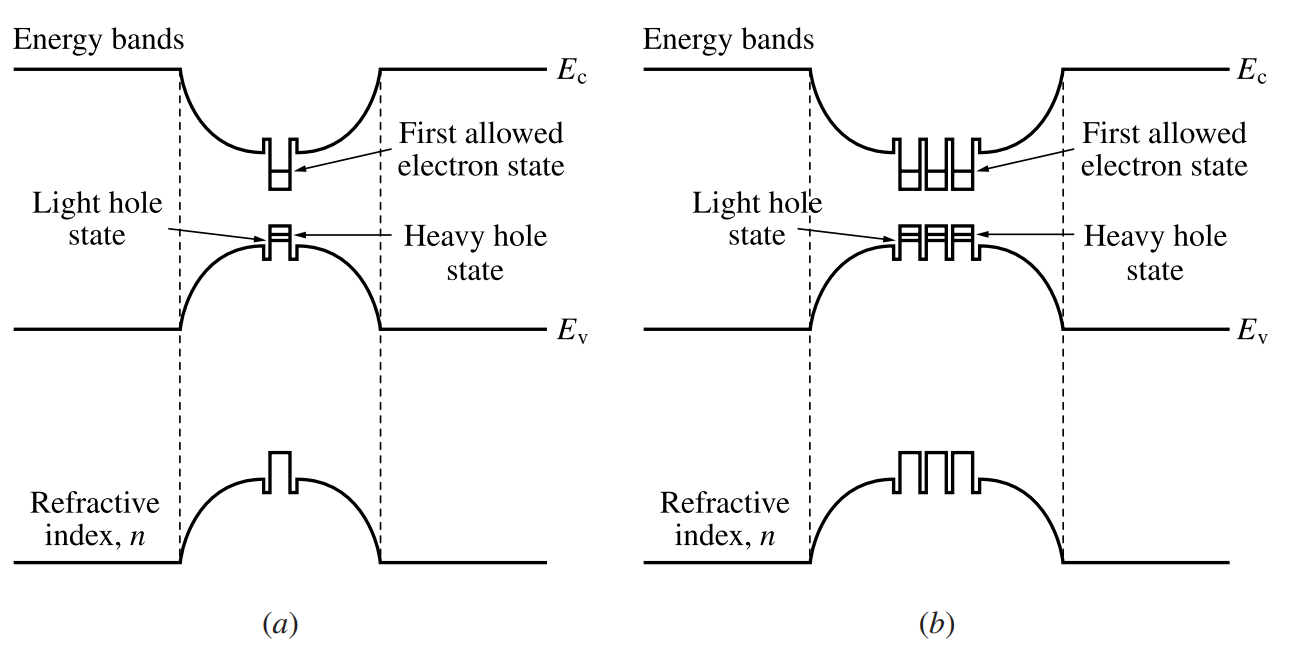Figure 13-14.  Energy bands and refractive index profiles of graded-index separate confinement heterostructures (GRIN-SCH) with (a) a single quantum well and (b) multiple quantum wells.

The next tutorial covers the topic of lateral structures of semiconductor junctions